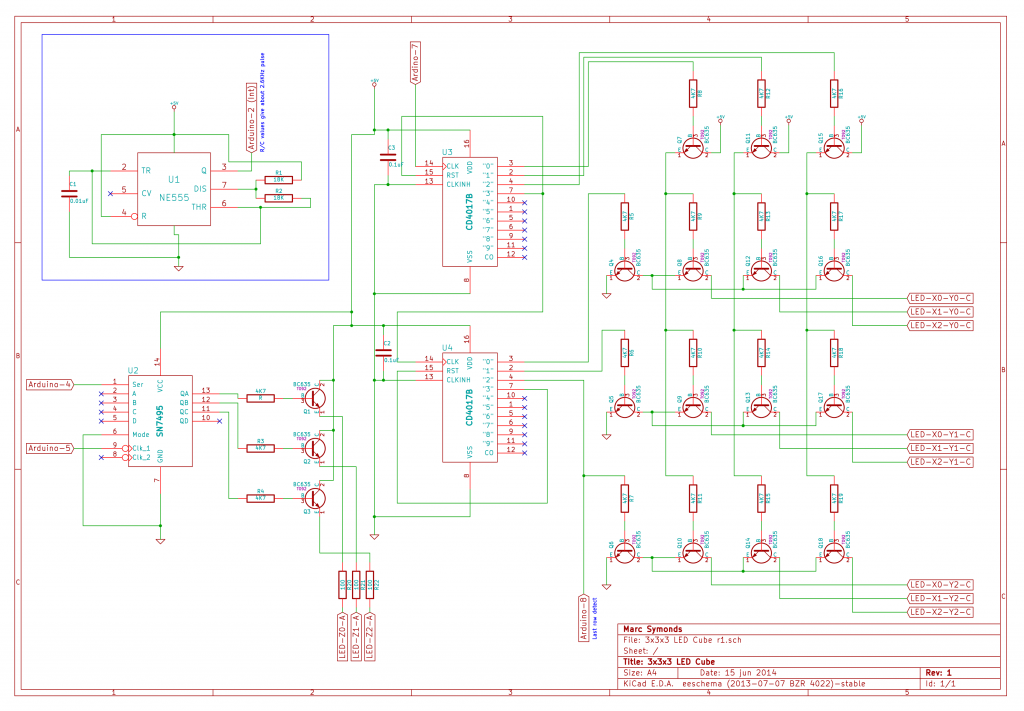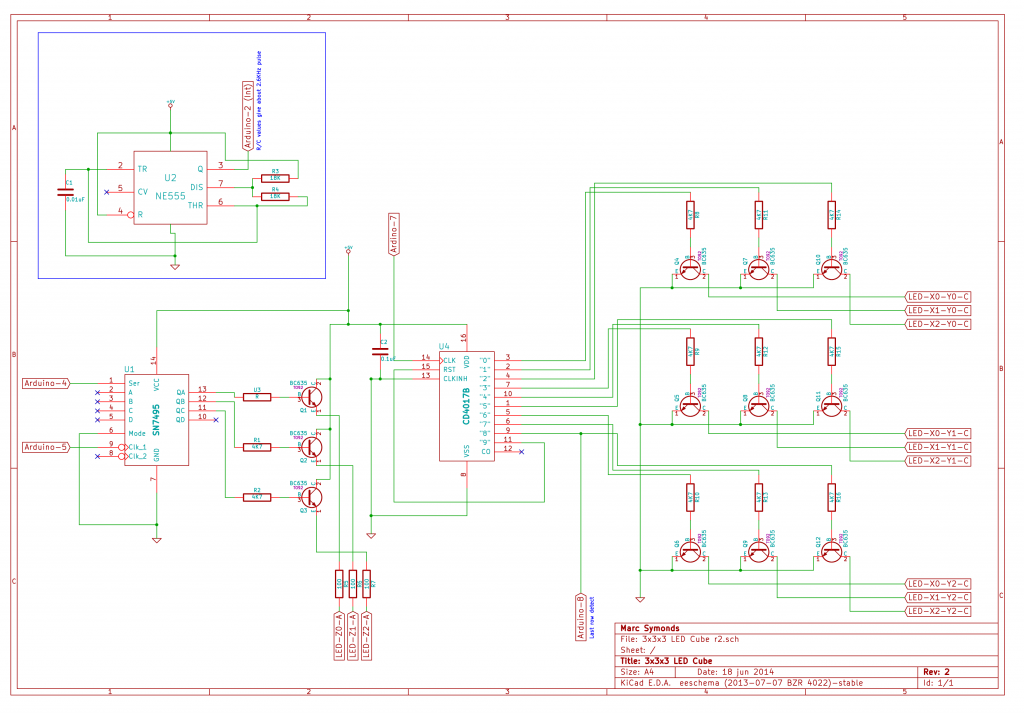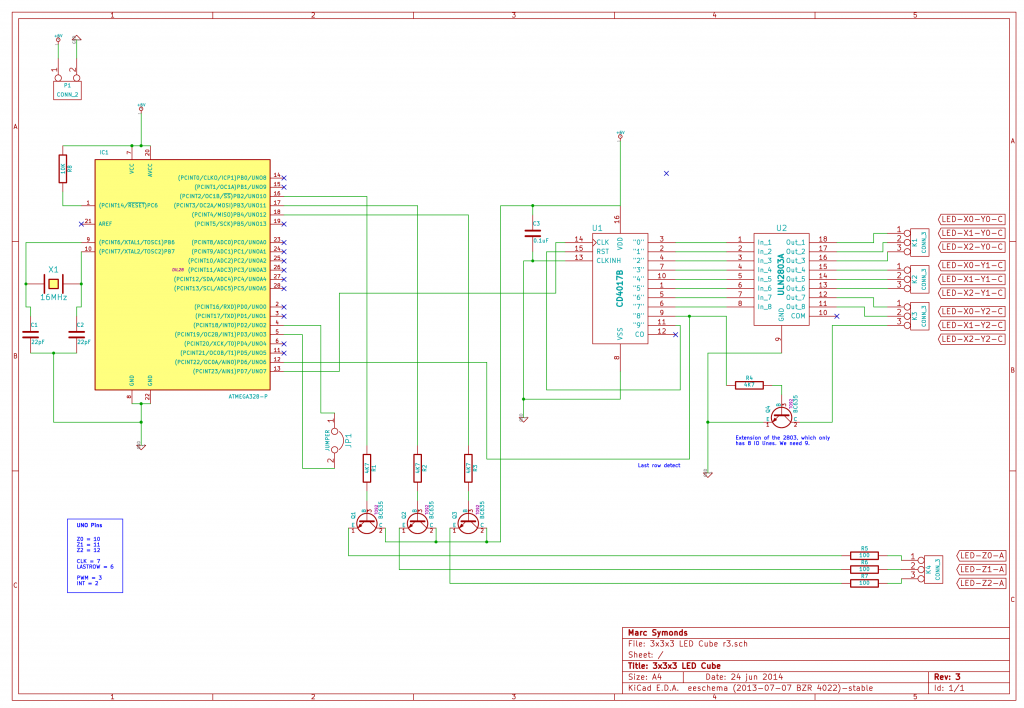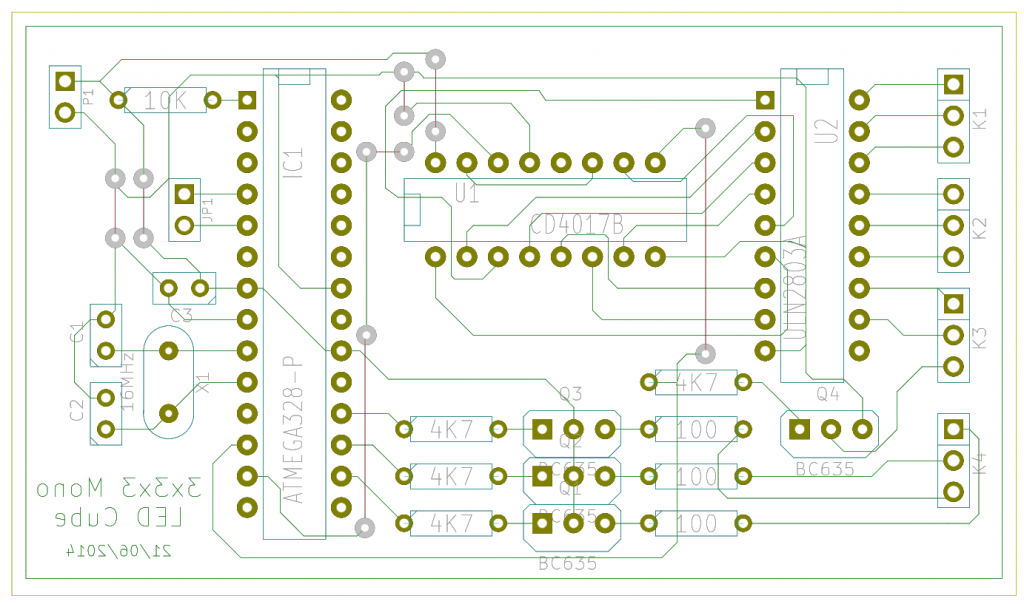MVFlashy.cpp

/* Project: 3x3x3 Mono LED Cube (r3).
* File: MVFlashy.cpp
* Description:
*     Movie -- mv_Flashy
*         Fades all LEDs in and out at random speeds.
*
* Copyright (C) 2014 Marc Symonds
* All rights reserved.
*
* This software may be used and redistribtued, with or without
* modification, as long as it is understood that this software
* is provided as-is without any explicit or implied warranties
* of merchantablity or fitness of purpose.
*/

#include "Arduino.h"
#include "Movies.h"
#include "DisplayFrame.h"

void mv_Flashy()
{
unsigned long ts;
byte y;
Light lights;

for (y = 0 ; y < 27 ; y++)
{
lights[y].speed = random(50, 200);
lights[y].up = true;
lights[y].t = millis() + lights[y].speed;
}

ts = millis() + DEF_MOVIE_TIME;

y = 0;
while (millis() < ts)
{
if (millis() > lights[y].t)
{
displayFrame[y] += (lights[y].up ? 1 : -1);
if (displayFrame[y] == 0 || displayFrame[y] == 7)
lights[y].up = !lights[y].up;

lights[y].t = millis() + lights[y].speed;
}

if (++y > 26)
y = 0;
}

}

MVEdgeTumble.cpp

/* Project: 3x3x3 Mono LED Cube (r3).
* File: MVEdgeTumble.cpp
* Description:
*     Movie -- mv_EdgeTumble
*         LEDs are lit along one edge, and then it "tumbles" from
*         edge to edge randomly. The LED in the center is faded
*         in and out.
*
* Copyright (C) 2014 Marc Symonds
* All rights reserved.
*
* This software may be used and redistributed, with or without
* modification, as long as it is understood that this software
* is provided as-is without any explicit or implied warranties
* of merchantablity or fitness of purpose.
*/

#include "Arduino.h"
#include "Movies.h"
#include "DisplayFrame.h"

const byte TUMBLESPEED = 25;
const byte TUMBLEDELAY = 150;
const byte FLASHERSPEED = 50;

int getN(byte, byte);

int m = {
{{1, 0}, {2, 0}},
{{1, 0}, {2, 1}},
{{1, 1}, {2, 1}},
{{1, 1}, {2, 2}},
{{1, 1}, {1, 2}},
{{0, 1}, {1, 2}},
{{0, 1}, {0, 2}}
};

unsigned long ft = 0;
boolean flashUp = true;
boolean flashOn = true;

void delayAndFlash(int d)
{
unsigned long t, ct;
byte a;

t = millis() + d;

do
{
ct = millis();

if (ct > ft)
{
a = displayFrame;
if (flashUp)
{
if (flashOn)
if (++a >= MAXBRIGHTNESSLEVEL)
flashUp = false;
}
else
{
if (--a < 1)
flashUp = true;
}
displayFrame = a;

ft = ct + FLASHERSPEED;
}
} while (ct < t);
}

void mv_EdgeTumble()
{
unsigned long ts;
byte a, b, f, l;
int nx, ny, nz;
int px, py, pz;
byte fn, fd;
Point p, v;

ft = millis() + 1000;
flashUp = (displayFrame < MAXBRIGHTNESSLEVEL);
flashOn = true;

ts = millis() + (DEF_MOVIE_TIME * 2);
l = 7;

// Random start point.

a = random(0, 8);
p.Set((a & 1) * 2, (a & 2), (a & 4) / 2);

// Random starting direction.

nx = 0;
ny = 0;
nz = 0;
switch(random(0, 3))
{
case 0:
nx = (p.x == 0) ? 1 : -1;
break;

case 1:
ny = (p.y == 0) ? 1 : -1;
break;

case 2:
nz = (p.z == 0) ? 1 : -1;
break;
}

// Draw initial line.

for (a = 1 ; a < 3 ; a++)
p[a].Set(p.x+(nx*a), p.y+(ny*a), p.z+(nz*a));

displayFrame[p.Pos()] = 7;
displayFrame[p.Pos()] = 7;
displayFrame[p.Pos()] = 7;

while (l > 0)
{
if (millis() > ts)
{
--l;
flashOn = false;
}

px = 0;
py = 0;
pz = 0;

fn = 1;
fd = 1;

switch(random(0, 2))
{
case 0:
px = (p.x == 0) ? 1 : -1;

if (nx != 0 || nz != 0)
pz = (p.z == 0) ? 1 : -1;

if (ny != 0)
py = (p.y == 0) ? 1 : -1;

if (ny != 0 || nz != 0)
{
fn = 5;
fd=255;
}
break;

case 1:
py = (p.y == 0) ? 1 : -1;

if (nx != 0)
px = (p.x == 0) ? 1 : -1;

if (ny != 0 || nz != 0)
pz = (p.z == 0) ? 1 : -1;

if (nz != 0)
{
fn = 5;
fd=255;
}
break;
}

displayFrame[p.Pos()] = l;

for (f = 0 ; f < 6 ; f++, fn += fd)
{
for (a = 1, b = 0 ; a < 3 ; a++, b++)
{
displayFrame[p[a].Pos()] = 0;

if (px == 0)
{
p[a].y = p.y + (m[fn][b] * py);
p[a].z = p.z + (m[fn][b] * pz);
}
else if (py == 0)
{
p[a].x = p.x + (m[fn][b] * px);
p[a].z = p.z + (m[fn][b] * pz);
}
else
{
p[a].x = p.x + (m[fn][b] * px);
p[a].y = p.y + (m[fn][b] * py);
}

displayFrame[p[a].Pos()] = l;
}

delayAndFlash(TUMBLESPEED);
}

v = p;
p = p;
p = v;

nx = getN(p.x, p.x);
ny = getN(p.y, p.y);
nz = getN(p.z, p.z);

delayAndFlash(TUMBLEDELAY);
}

}

int getN(byte n1, byte n2)
{
if (n1 == n2)
return 0;
else if (n1 < n2)
return 1;
else
return -1;
}

MVEdgeChase.cpp

/* Project: 3x3x3 Mono LED Cube (r3).
* File: MVEdgeChase.cpp
* Description:
*     Movie -- mv_EdgeChase
*         LEDs are lit along an edge from one corner to another
*         at a random speed. After a random delay another edge
*         is lit. If the lighting of an edge runs in to an already
*         lit LED, then the display is cleared and it starts again.
*
*     Movie -- mv_EdgeChase2
*         LEDs are lit along an edge from one corner to another,
*         but as it moves along, each previous LED is dimmed
*         until it goes out.
*
* Copyright (C) 2014 Marc Symonds
* All rights reserved.
*
* This software may be used and redistributed, with or without
* modification, as long as it is understood that this software
* is provided as-is without any explicit or implied warranties
* of merchantablity or fitness of purpose.
*/

#include "Arduino.h"
#include "Movies.h"
#include "DisplayFrame.h"

void mv_EdgeChase()
{
unsigned long ts, t;
byte a, b, x, y, z;
int nx, ny, nz;
boolean r = false;
int d1, d2;

ts = millis() + DEF_MOVIE_TIME;

d1 = random(40, 120);
d2 = random(20, 60);

a = random(0, 8);

x = (a & 1) * 2;
y = (a & 2);
z = (a & 4) / 2;

a = 3;

displayFrame[POS(x, y, z)] = MAXBRIGHTNESSLEVEL;

while (millis() < ts)
{
t = millis() + d1;

nx = 0;
ny = 0;
nz = 0;

do
{
b = random(0, 3);
} while (a == b);

a = b;

switch(a)
{
case 0:
nx = (x == 0) ? 1 : -1;
break;

case 1:
ny = (y == 0) ? 1 : -1;
break;

case 2:
nz = (z == 0) ? 1 : -1;
break;
}

while (millis() < t)
;

if (r)
df_clear();

displayFrame[POS(x, y, z)] = 4;
displayFrame[POS(x+nx,y+ny,z+nz)] = MAXBRIGHTNESSLEVEL;

delay(d2);

displayFrame[POS(x+nx,y+ny,z+nz)] = 4;
r = (displayFrame[POS(x+nx+nx,y+ny+ny,z+nz+nz)] != 0);
displayFrame[POS(x+nx+nx,y+ny+ny,z+nz+nz)] = MAXBRIGHTNESSLEVEL;

delay(d2);

displayFrame[POS(x+nx,y+ny,z+nz)] = 1;

x = x + nx + nx;
y = y + ny + ny;
z = z + nz + nz;
}

df_clear();
}

void mv_EdgeChase2()
{
unsigned long ts, t;
byte a, b, d, i, j, k, x, y, z;
int nx, ny, nz;
byte ss, sl;

ts = millis() + DEF_MOVIE_TIME;

d = random(50, 200);

a = random(0, 8);

x = (a & 1) * 2;
y = (a & 2);
z = (a & 4) / 2;
points.Set(x, y, z);
ss = 0;
sl = 1;

a = 3;

displayFrame[points.Pos()] = MAXBRIGHTNESSLEVEL;

t = millis() + d;

while (millis() < ts)
{
nx = 0;
ny = 0;
nz = 0;

do
{
b = random(0, 3);
} while (a == b);

a = b;

switch(a)
{
case 0:
nx = (x == 0) ? 1 : -1;
break;

case 1:
ny = (y == 0) ? 1 : -1;
break;

case 2:
nz = (z == 0) ? 1 : -1;
break;
}

for (i = 0 ; i < 2 ; i++)
{
while (millis() < t)
;

j = ss;
for (k = 0 ; k < sl ; k++)
{
if (displayFrame[points[j].Pos()] > 0)
--displayFrame[points[j].Pos()];

if (j == 0)
j = 7;
else
--j;
}

if (++ss > 7)
ss = 0;

if (sl < 8)
++sl;

x = x + nx;
y = y + ny;
z = z + nz;

points[ss].Set(x, y, z);
displayFrame[points[ss].Pos()] = MAXBRIGHTNESSLEVEL;

t = millis() + d;
}
}

df_clear();
}

MVCounter.cpp

/* Project: 3x3x3 Mono LED Cube (r3).
* File: MVCounter.cpp
* Description:
*     Movie -- mv_Counter
*         LED on bottom plane moves around the edge of the
*         plane. On each complete circuit, the LED on the
*         plane above moves along one, and when that has done
*         a complete circuit, the led on the top plane moves
*         along one.
*
* Copyright (C) 2014 Marc Symonds
* All rights reserved.
*
* This software may be used and redistributed, with or without
* modification, as long as it is understood that this software
* is provided as-is without any explicit or implied warranties
* of merchantablity or fitness of purpose.
*/

#include "Arduino.h"
#include "Movies.h"
#include "DisplayFrame.h"

struct Item
{
public:
byte x;
byte d;

Item() : x(0), d(0) {};

boolean inc()
{
boolean o = false;

switch(d)
{
case 0:
if (++x == 2)
d = 1;
break;

case 1:
x += 9;
if (x > 18)
d = 2;
break;

case 2:
if (--x == 18)
d = 3;
break;

case 3:
x -= 9;
if (x < 9)
{
d = 0;
o = true;
}
break;
}

return o;
}
};

void mv_Counter()
{
Item i;
unsigned long ts, t;
boolean o;
int del;

i.x = 0;
i.x = 0;
i.x = 0;

displayFrame[i.x] = 7;
displayFrame[i.x + 3] = 7;
displayFrame[i.x + 6] = 7;

delay(1000);

del = random(20, 70);

ts = millis() + (DEF_MOVIE_TIME * 2);

while (millis() < ts)
{
t = millis() + del;

displayFrame[i.x] = 0;
o = i.inc();
displayFrame[i.x] = 7;
if (o)
{
displayFrame[i.x + 3] = 0;
o = i.inc();
displayFrame[i.x + 3] = 7;
if (o)
{
displayFrame[i.x + 6] = 0;
i.inc();
displayFrame[i.x + 6] = 7;
}
}

while (millis() < t)
;
}

delay(500);
}

MVAllOn.cpp

/* Project: 3x3x3 Mono LED Cube (r3).
* File: MVAllOn.cpp
* Description:
*     Movie -- mv_AllOn
*         Turn all LEDs on for a period, then fade out.
*
* Copyright (C) 2014 Marc Symonds
* All rights reserved.
*
* This software may be used and redistributed, with or without
* modification, as long as it is understood that this software
* is provided as-is without any explicit or implied warranties
* of merchantablity or fitness of purpose.
*/

#include "Arduino.h"
#include "Movies.h"
#include "DisplayFrame.h"

void mv_AllOn()
{
unsigned long ts;

ts = millis() + (DEF_MOVIE_TIME / 10);

df_allOn();

while (millis() < ts)
;

delay(500);
}

DisplayFrame.h

/* Project: 3x3x3 Mono LED Cube (r3).
* File: DisplayFrame.h
* Description: Header file for DisplayFrame.cpp.
*
* Copyright (C) 2014 Marc Symonds
* All rights reserved.
*
* This software may be used and redistributed, with or without
* modification, as long as it is understood that this software
* is provided as-is without any explicit or implied warranties
* of merchantablity or fitness of purpose.
*/

#ifndef DisplayFrame_h
#define DisplayFrame_h

#include "Arduino.h"

typedef byte (*POSTransFPtr)(byte, byte, byte);

byte POSTrans0(byte, byte, byte);
byte POSTrans1(byte, byte, byte);
byte POSTrans2(byte, byte, byte);
byte POSTrans3(byte, byte, byte);
byte POSTrans4(byte, byte, byte);
byte POSTrans5(byte, byte, byte);
byte POSTrans6(byte, byte, byte);
byte POSTrans7(byte, byte, byte);

POSTransFPtr GetPOSTrans(byte);

#define POS(x, y , z)  (((y)*9)+((x)*3)+(z))

struct DisplayParameters
{
public:
byte subFrame;
byte subFrameBit;
byte x;

DisplayParameters() : subFrame(0), subFrameBit(1), x(0) {};
};

struct Point
{
public:
byte x;
byte y;
byte z;

Point() : x(0), y(0), z(0) {};
Point(byte a, byte b, byte c) : x(a), y(b), z(c) {};

void Set(byte a, byte b, byte c)
{
x = a;
y = b;
z = c;
}

byte Pos()
{
return POS(x, y, z);
}
};

class Light
{
public:
byte speed;
boolean up;
unsigned long t;
};

const byte MAXBRIGHTNESSLEVEL = 7;

extern byte displayFrame;
extern Point points;
//extern const byte brightnessSubFrames;

typedef boolean (*df_processAllFunc)(int *, byte);

void df_init();
void updateDisplay();
void df_allOn();
void df_clear();
boolean df_processAll(df_processAllFunc, int *);

void df_DisplayMonoFrame(POSTransFPtr, unsigned long);

#endif

DisplayFrame.cpp

/* Project: 3x3x3 Mono LED Cube (r3).
* File: DisplayFrame.cpp
* Description: Functions for updating the LED cube.
*
* Copyright (C) 2014 Marc Symonds
* All rights reserved.
*
* This software may be used and redistributed, with or without
* modification, as long as it is understood that this software
* is provided as-is without any explicit or implied warranties
* of merchantablity or fitness of purpose.
*/

#include "Arduino.h"
#include "DisplayFrame.h"
#include "DirectIO.h"
#include "ShiftReg_7495.h"
#include "Counter_4017.h"

const byte brightnessSubFrames[] = {B00000000, B00000001, B01001001, B01010101, B01011101, B01110111, B11110111, B11111111};

byte displayFrame;

Point points; // An array of points that animations can use.

volatile DisplayParameters dispParams;

ShiftReg_7495 DisplayZ(4, 10, 11, 12); // Parallel mode: Clock on pin 4, A on pin 10, B on pin 11 and C on pin 12. NOT ACTUALLY USING PIN 4 - PINS 10, 11 and 12 WILL DRIVE THE DISPLAY DIECTLY.
Counter_4017 DisplayXY(7, 6); // Clock pin 7, last element detect pin 6.

// Used for timing metrics in the updateDisplay function.

volatile boolean gotupt = false;
volatile unsigned int upt = 0;
volatile unsigned int upc = 0;

void df_init()
{
df_clear();
attachInterrupt(0, updateDisplay, RISING); // Interrupt 0 is on pin 2 (DISPLAYCLOCKPIN).
}

// Called by interrupt 0.
// Displays a 3 LED segment on each interrupt.

void updateDisplay()
{
byte b, c, d;
unsigned long st = 0, et = 0;

// Timing metrics.

if (gotupt == false)
{
st = micros(); // micros() still works in an interrupt - apparently.
}

DisplayZ.clear();    // Clear data lines.
DisplayXY.clock();   // Trigger 4017 - move to the next row to display on.

// Move to next row to display.

dispParams.x += 3;
if (dispParams.x > 26)
{
dispParams.x = 0;

// Subframe for dimming LED.

if (++dispParams.subFrame > MAXBRIGHTNESSLEVEL)
{
dispParams.subFrame = 0;
dispParams.subFrameBit = 1;
}
else
dispParams.subFrameBit <<= 2;
}

// Set bits to be displayed.

c = dispParams.x;
for (b = 0 ; b < 3 ; b++)
{
DisplayZ.setBit(b, (brightnessSubFrames[displayFrame[c]] & dispParams.subFrameBit) != 0);
++c;
}

/*
This loop is a delay to allow the triggering of the 4017 (DisplayXY) to propagate.
The 4017 can take up to 530ns from tigger to output at 5V, according to data sheet.

Without this delay, we will see the new data being applied to the current data lines
before the 4017 has acted. So you get a ghost of the next set of data on the current
leds.
*/

for (d = 0 ; d < 9 ; d++)

// Display the bits (LEDs).

DisplayZ.send();

// Timing metrics.

if (gotupt == false && st > 0)
{
et = micros();
upt = et - st;
st = 0;
gotupt = true;
}

++upc;
}

void df_allOn()
{
for (byte i = 0 ; i < 27 ; i++)
displayFrame[i] = MAXBRIGHTNESSLEVEL;
}

void df_clear()
{
for (byte i = 0 ; i < 27 ; i++)
displayFrame[i] = 0;
}

{
int changed = 0;

{
changed = 0;

delay(delayTime);
}

}

{
if (displayFrame[cell] > 0)
{
displayFrame[cell] -= 1;
*data += 1;
}

return true;
}

boolean df_processAll(df_processAllFunc func, int *data)
{
byte x;
boolean success = true;

for (x = 0 ; x < 27 ; x++)
{
if (!func(data, x))
{
success = false;
continue;
}
}

return success;
}

POSTransFPtr GetPOSTrans(byte t)
{
switch(t)
{
case 1:
return POSTrans1;
break;

case 2:
return POSTrans2;
break;

case 3:
return POSTrans3;
break;

case 4:
return POSTrans4;
break;

case 5:
return POSTrans5;
break;

case 6:
return POSTrans6;
break;

case 7:
return POSTrans7;
break;

default:
return POSTrans0;
break;
}
}

byte POSTrans0(byte x, byte y, byte z)
{
return(POS(x, y, z));
}

byte POSTrans1(byte x, byte y, byte z)
{
return(POS(2-x, y, z));
}

byte POSTrans2(byte x, byte y, byte z)
{
return(POS(x, 2-y, z));
}

byte POSTrans3(byte x, byte y, byte z)
{
return(POS(2-x, 2-y, z));
}

byte POSTrans4(byte x, byte y, byte z)
{
return(POS(x, y, 2-z));
}

byte POSTrans5(byte x, byte y, byte z)
{
return(POS(2-x, y, 2-z));
}

byte POSTrans6(byte x, byte y, byte z)
{
return(POS(x, 2-y, 2-z));
}

byte POSTrans7(byte x, byte y, byte z)
{
return(POS(2-x, 2-y, 2-z));
}

// Each bit in "box" represents an LED in the display; 0=Off, 1 = On.

void df_DisplayMonoFrame(POSTransFPtr trans, unsigned long box)
{
register byte x = 0, y = 0, z = 0;

for (int i = 0 ; i < 27 ; i++)
{
displayFrame[trans(x, y, z)] = (box & 1) == 1 ? MAXBRIGHTNESSLEVEL : 0;
box >>= 1;
if (++x > 2)
{
x = 0;
if (++y > 2)
{
y = 0;
++z;
}
}
}
}

_3x3x3_Mono.ino

/* Project: 3x3x3 Mono LED Cube (r3).
* File: _3x3x3_Mono.ino
* Description: 3x3x3 mono LED cube using Arduino UNO.
*
* Copyright (C) 2014 Marc Symonds
* All rights reserved.
*
* This software may be used and redistributed, with or without
* modification, as long as it is understood that this software
* is provided as-is without any explicit or implied warranties
* of merchantablity or fitness of purpose.
*/

#include "DirectIO.h"
#include "Counter_4017.h"
#include "ShiftReg_7495.h"

#include "DisplayFrame.h"
#include "Movies.h"

const int DISPLAYCLOCKPIN = 2; // Must be pin 2, for interrupt 0.
const int CLOCKGENPIN = 3; // Pin that will generate the interrupt pulse. Could use external pulse generator instead.

void setup()
{
pinMode(DISPLAYCLOCKPIN, INPUT);
pinMode(CLOCKGENPIN, OUTPUT);

// Set PWM on pin 3 to about 3.9KHz and start it.
TCCR2B = (TCCR2B & B11111000) | B010;
analogWrite(CLOCKGENPIN, 127);

// Seed the random number generator.

// Initialise the displayFrame functions.
df_init();
}

void loop()
{
static byte a = 255;
byte b;

do
{
b = random(0, 14);
} while (b == a);
a = b;

switch(b)
{
case 0:
mv_Boxy();
break;

case 1:
mv_EdgeChase2();
break;

case 2:
mv_Snake();
break;

case 3:
mv_Counter();
break;

case 4:
mv_EdgeTumble();
break;

case 5:
mv_Flashy();
break;

case 6:
mv_AllOn();
break;

case 7:
mv_Rain();
break;

case 8:
mv_EdgeChase();
break;

case 9:
mv_Jitter();
break;

case 10:
mv_Pulse();
break;

case 11:
mv_TwinkleAll();
break;

case 12:
mv_Lightning();
break;

default:
mv_Glitter();
break;
}
}

3x3x3 Mono LED Cube

Previously, I have used stripboard for making electronic circuits. I was watching some episodes of EEVBlog on YouTube, and in one episode he looked at an open source application suite call KiCad which allows you to design electronic schematics and PCBs. So I thought I would also have a go at creating my own PCB rather than use stripboard.

Altough I still used stripboard for prototyping because it's quicker and easier.

I wanted to have the ability to have different brightness levels, but I didn't think the PWM feature of the UNO would work very well since each LED would only be on for a fraction of a second; If I wanted 30 frames per second, there are 9 columns to scan through which means 270 updates per second. So I thought of using a higher scan rate and have each LED during each frame scan depending on its brightness level. I decided on 8 levels of brightness, including off, which meant I would need a scan rate of 270*8 = 2160 updates per second.

I was new to the ATMEGA328-P (Arduino UNO), and wasn't aware of the options I had with it, so in my first test I used a 555 timer to provide a pulse. The R/C values I used gave me a pulse of about 2.6KHz. The pulse is used to raise an interrupt in the 328-P, and each interrupt forces the next column of LEDs to be displayed. I discovered later that I could get rid of the 555 and use one of the PWM pins on the 328-P to provide the pulse. The range of PWM options is limited, but I could get a 3.9KHz pulse which was a bit faster than I needed, but it worked,

Revision 1

The first revision is a test of how I thought a larger LED cube could be made.This version uses two 4017 decade counters to provide the X/Y scanning. These drive the transistors in the array which ground each column of 3 LEDs. The 4017's are slaved together; when the first one gets to the fourth row, it resets itself and then clocks the second 4017. When the second 4017 gets to the fourth row, it resets itself. Each 4017 drives three transistors (X and Y), which together then turn on one of the transistors in the 3×3 array.

The 555 timer is used to create a continuous pulse to the Arduino. This pulse is used to raise an interrupt. The interrupt calls the display routine which draws the next "column" of LEDs. The cube consitst of 9 "columns", and I wanted at least a 30fps display rate. I also wanted to have some sort brightness level; I decided on 8 levels. This meant I needed a pulse frequency of at least 30*9*8 = 2160 hertz. The capacitor and resistor values I used gave about 2.6KHz.

Revision 2

The first revision was a test of using the 4017s and an array of transistors to control a X/Y matrix. This second revision removes most of the unncessary components and just makes a 3x3x3 cube.I got rid of one of the 4017s, and also the X/Y control transistor. The 4017 could directly drive the X/Y column transistors.

Revision 3

Removed some more unnecessary componants. I can get rid of the 555 and just use one of the PWM pins on the Arduino to provide the interrupt pulse. I can also get rid of the shift register and just use 3 pins from the Arudino directly. I can also replace 8 of the transistors in the 3×3 array with a ULN2803A, which is an 8 way Darlington array.Finished 3x3x3 LED Cube in action using a home made PCB, except for the LEDs which are on a piece of stripboard.

PCB Layout (component side):-Completed PCB:-